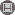دوره 8، شماره 2 - ( 7-1392 )                   جلد 8 شماره 2 صفحات 49-55 | برگشت به فهرست نسخه ها

Download citation:
BibTeX | RIS | EndNote | Medlars | ProCite | Reference Manager | RefWorks
Send citation to:Alikhani S. On the domination polynomials of non P4-free graphs. IJMSI. 2013; 8 (2) :49-55
URL: http://ijmsi.ir/article-1-504-fa.html
On the domination polynomials of non P4-free graphs. مجله علوم ریاضی و انفورماتیک. 1392; 8 (2) :49-55

چکیده:

A graph \$G\$ is called \$P_4\$-free, if \$G\$ does not contain an induced subgraph \$P_4\$. The domination polynomial of a graph \$G\$ of order \$n\$ is the polynomial \$D(G,x)=sum_{i=1}^{n} d(G,i) x^{i}\$, where \$d(G,i)\$ is the number of dominating sets of \$G\$ of size \$i\$. Every root of \$D(G,x)\$ is called a domination root of \$G\$. In this paper we state and prove formula for the domination polynomial of non \$P_4\$-free graphs. Also, we pose a conjecture about domination roots of these kind of graphs.

نوع مطالعه: پژوهشي | موضوع مقاله: عمومى

ارسال نظر درباره این مقاله : نام کاربری یا پست الکترونیک شما:کلیه حقوق این وب سایت متعلق به نشریه علوم ریاضی و انفورماتیک می باشد.

طراحی و برنامه نویسی : یکتاوب افزار شرق

© 2021 CC BY-NC 4.0 | Iranian Journal of Mathematical Sciences and Informatics

Designed & Developed by : Yektaweb